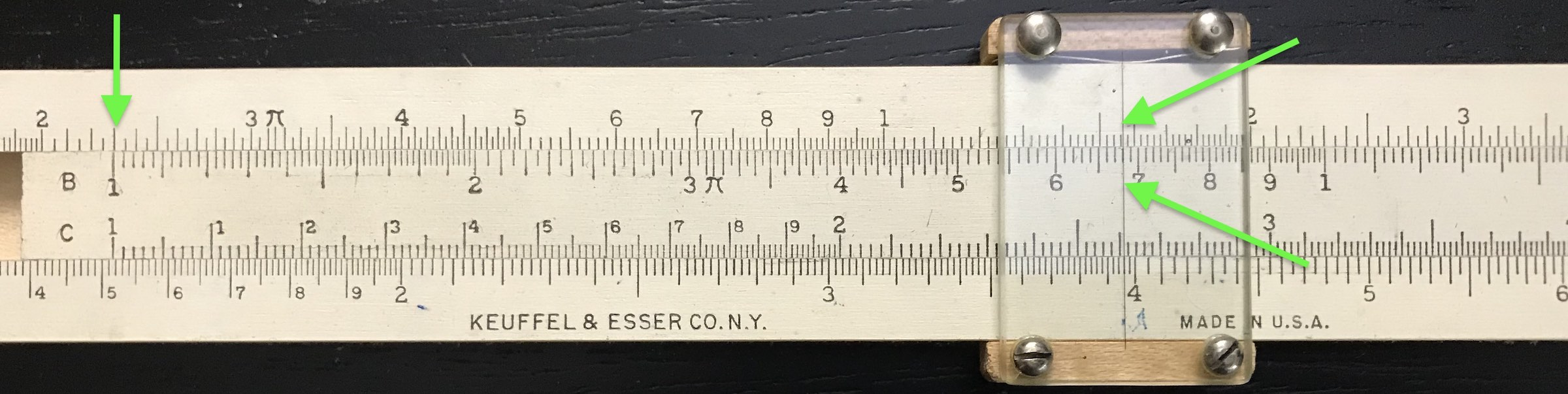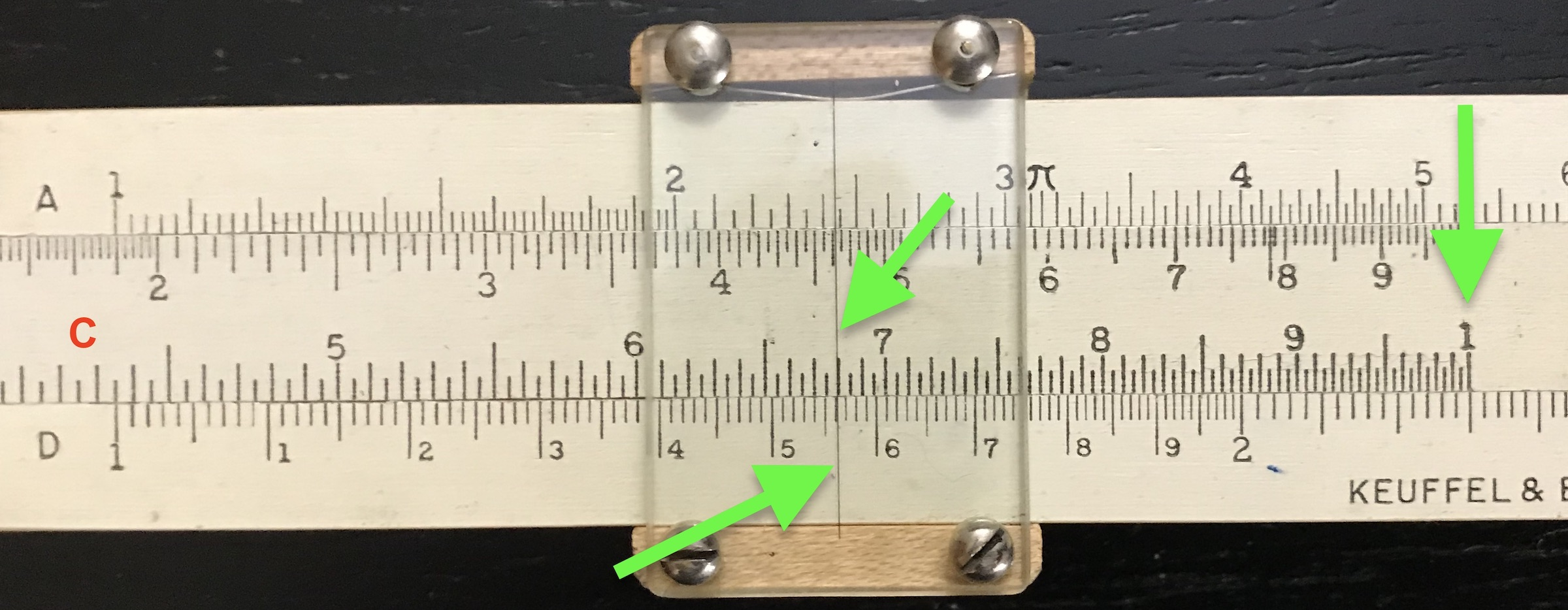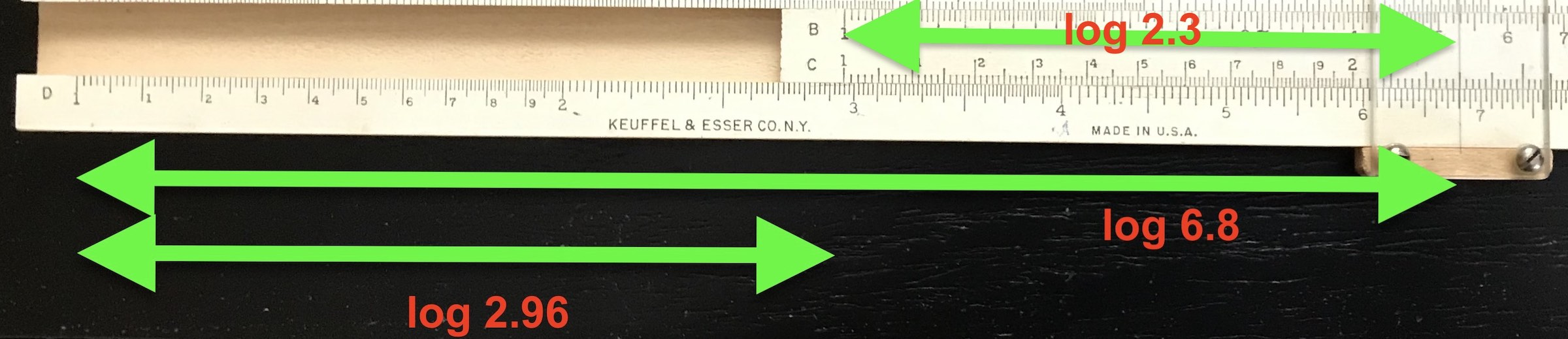## 3.1 Basic Multiplication and Division

To multiply two numbers we can use one set of scales, either A and B, or C and D. Let’s try A and B first. Suppose we want to multiply two numbers, each of which are between 1 and 10: Let’s say $$2.3 \times 6.8$$. To do so, …

• slide the 1 on the B scale to line up with 2.3 on the A scale
• slide the cursor to line up with 6.8 on the B scale
• follow the cursor line to view the number under the cursor on the A scale; this is the final answer: 15.6 (to three digits)A Multiplication Example.

If we zoom out for a moment, we can see what is going on. To find the product, we are actually adding the logarithms of the two numbers in question, and finding out which number has a logarithm equal to that sum.Addition of logarithmic distances.

Note that it may be hard to tell on the A scale whether the answer is closer to 15.5 or 15.6, etc., due to the granularity of the scale one is reading, especially for numbers on the right-hand end of the scale. So, one may have to “guess” at that third decimal place.

And that’s one reason for the C/D scales. Since one decade (factor of 10) on these logarithmic scales is twice as long as those on the A/B scales, the C/D scales have more accuracy and hence might really be important for getting that third digit correct. In general, the scale or scales on the slide rule that have the greatest length for a single factor of 10 are called the C/D scales. Some rules, called long-scale rules, can have C/D scales that are 50 cm (20 inches) rather than 25 cm (10 inches), and some can have scales wound up in circular, spiral or helical forms which can give overall scale lengths much longer for higher accuracy. But 25 cm is generally considered the “standard” length and gives a typical accuracy of about three digits.8 Even smaller lengths (which can fit in a pocket, for instance) can be found, but while convenient they are seldom as accurate. (See Non-Linear and Long-Scale Rules for examples of long-scale rules, and Pocket Rules for examples of smaller-scale rules.)

So, let’s do the same multiplication calculation using the C/D scales:

• slide the 1 on the C scale to line up with 2.3 on the D scale
• slide the cursor to line up with 6.8 on the D scale. $$~~~$$ Oops! What just happened?
• Because the C/D scales only go from 1 to 10, the above operation takes us off scale!
• No problem; since every decade of a logarithmic scale has the same spacing of divisions, we simply need to imagine starting the problem from the left and then keep track of our factors of 10. So, let’s repeat the operation, but this time …
• slide the 10 on the C scale to line up with 2.3 on the D scale instead of using the 1
• slide the cursor (to the left!) to line up with the 6.8 on the C scale
• now, follow the cursor line to view the number under the cursor on the D scale; this is the answer (within a factor of ten).

You’ll see that the answer that you read off will be 1.56 on the D scale. But because we lined up with the right-hand-edge of the scale rather than the left, there is an extra factor of ten involved. Also, since you were multiplying 2.3 and 6.8 you know that the answer should be something closer to 15, so the correct answer must be 15.6.Using the C/D Scales.

Two things to learn here: The C/D scales give more accuracy in most cases than the A/B scales. So, they’d be the “go-to” scales. Also, it is always important to take a guess at what the answer should be so that one gets the right power of ten in the end. The slide rule will keep track of the digits in the answer; the user will have to keep track of the powers of 10.

General Rule: To multiply, find the value of the first number on the D scale, then line up a “1” on the C scale with this first number, using whichever end of the C scale gives access to the second number (also on the C scale). Slide the cursor to the second number as found on the C scale. Follow the cursor line to read off the answer on the D scale directly below.

What about division? Let’s take 6.8 divided by 2.3 as our example. We follow these steps (using C/D):

• slide the cursor to 6.8 on the D scale
• slide the 2.3 on the C scale to line up with 6.8 on the D scale
• slide the cursor to 1 on the C scale
• follow the cursor line to view the number under the cursor on the D scale; this is the answer, again, within a factor of ten. In our case here, it is the answer, as 6.8/2.3 should be about 2.96.Division using the C/D Scales.

What just happened? We found 6.8 on the rule, noting that its distance from 1 on the rule is equal to $$\log 6.8$$. We then subtracted a distance that corresponds to $$\log 2.3$$. The result is given by a length corresponding to $$\log 2.96$$.

General Rule: To divide, line up the cursor with the numerator on the D scale; slide the C scale so that the denominator also lines up with the cursor; the answer will be on the D scale directly below the “1” on the C scale.

If you think about the above operations for a while, you should realize that you are either adding logarithms to perform multiplications, or subtracting logarithms to perform divisions. Once you realize that that is what you’re doing, then you’ll be able to perform sequences of multiplications and divisions with lightening speed.

As an illustration, let’s estimate the average speed in miles per hour of the earth in its orbit (radius = 93.0 million miles) about the sun using a slide rule. Set up the problem by gathering factors of ten as follows:

$v = \frac{2 \pi R}{T} = \frac{2 \pi ~ (93.0\times 10^6 ~ {\rm mi})} { 1~{\rm year} \times 365~{\rm day/year} \times 24 ~ {\rm hr/day} } = \frac{2 \times \pi \times 9.30 \times 10^7 ~ {\rm mi}} { 3.65 \times 2.4 \times 10^3 ~~{\rm hr}} \\ = \frac{2 \times \pi \times 9.30} { 2.4 \times 3.65} \times 10^4 ~ {\rm mi/hr}. \\$

It is good practice and often useful to order the numbers in the numerator and denominator such that factors of near-equal value are above/below each other. First, this practice gives you an early impression of what the result will be. From our numbers above, we see that multiplying and dividing these numbers should give us an answer somewhat less than 9. Also, by performing a multiplication followed by a division and then repeating using the numbers from left to right, this tends to keep the result fairly centered on the rule. So, here are the next steps using the slide rule:

• Move the cursor to 2 on the D scale
+ which, technically, is multiplying 1 by 2.
• Set the C scale to 2.4 at the cursor
+ thus dividing by 2.4, according to our rules; the answer thus far would be found on the D scale under the “1” on the C scale; but we don’t really need that answer at the moment.
• Move the cursor to $$\pi$$ (3.142) on the C scale
+ thus multiplying the previous result by $$\pi$$.
• Set the C scale to 3.65 at the cursor
+ thus dividing the previous result by 3.65.
• Move the cursor to 9.3 on the C scale
+ thus multiplying the previous result by 9.30.

The result is read on the D scale under the cursor; it should be about 6.67. This is consistent with our estimate that it be less than 9.

The FINAL answer is this number times $$10^4$$, or 66,700 mi/hr.

Compare with result from a computer calculation:

##  2*pi*93.0e6/24/365 = 66705.0494940299

1. The Englishman William Oughtred invented the slide rule, and the standard became a 10-inch rule – 10 inches for every factor of 10. Eventually, the more conventional standard became metric – 25 cm per factor of 10.↩︎